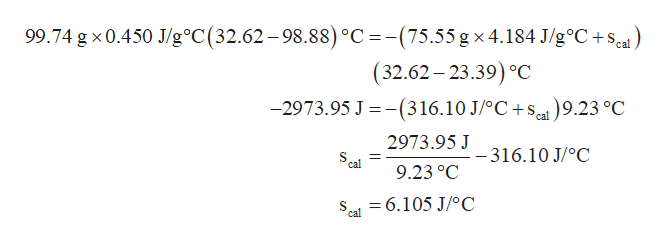# In the laboratory a "coffee cup" calorimeter, or constant pressure calorimeter, is frequently used to determine the specific heat of a solid, or to measure the energy of a solution phase reaction. A chunk of platinum weighing 19.07 grams and originally at 97.71 °C is dropped into an insulated cup containing 79.75 grams of water at 20.99 °C. In the laboratory a "coffee cup" calorimeter, or constant pressure calorimeter, is frequently used to determine the specific heat of a solid, or to measure the energy of a solution phase reaction. Since the cup itself can absorb energy, a separate experiment is needed to determine the heat capacity of the calorimeter. This is known as calibrating the calorimeter and the value determined is called the calorimeter constant. One way to do this is to use a common metal of known heat capacity.  In the laboratory a student heats 99.74 grams of iron to 98.88 °C and then drops it into a cup containing 75.55 grams of water at 23.39 °C. She measures the final temperature to be 32.62 °C. Using the accepted value for the specific heat of iron (See the References tool), calculate the calorimeter constant.

Question
97 views

In the laboratory a "coffee cup" calorimeter, or constant pressure calorimeter, is frequently used to determine the specific heat of a solid, or to measure the energy of a solution phase reaction.

A chunk of platinum weighing 19.07 grams and originally at 97.71 °C is dropped into an insulated cup containing 79.75 grams of water at 20.99 °C.

In the laboratory a "coffee cup" calorimeter, or constant pressure calorimeter, is frequently used to determine the specific heat of a solid, or to measure the energy of a solution phase reaction.

Since the cup itself can absorb energy, a separate experiment is needed to determine the heat capacity of the calorimeter. This is known as calibrating the calorimeter and the value determined is called the calorimeter constant.

One way to do this is to use a common metal of known heat capacity.  In the laboratory a student heats 99.74 grams of iron to 98.88 °C and then drops it into a cup containing 75.55 grams of water at 23.39 °C. She measures the final temperature to be 32.62 °C.

Using the accepted value for the specific heat of iron (See the References tool), calculate the calorimeter constant.

check_circle

Step 1

The relation between the amount of heat gained or lost (q) by a metal in a chemical process and the specific heat (s) of metal is given in expression (1). In this expression, m is the mass of the metal, s is the specific heat, T1 is the final temperature, and T2 is the final temperature.

Step 2

In the given experiment, the amount of heat released by iron is equal to the negative of the heat absorbed by water and the heat absorbed by calorimeter present in the system. Hence, the expression for calculating the heat capacity of calorimeter can be obtained by substituting the values of q from the equation (1).

Step 3

The value of heat capacity of calorimeter is cal...help_outlineImage Transcriptionclose99.74 g x 0.450 J/g°C(32.62-98.88) °C =-(75.55 gx 4.184 J/g°C+se (32.62-23.39) °C -2973.95 J -(316.10 J/°C+s 9.23 °C 2973.95 J -316.10 J/oC cal 9.23 °C =6.105 J/oC cal fullscreen

### Want to see the full answer?

See Solution

#### Want to see this answer and more?

Solutions are written by subject experts who are available 24/7. Questions are typically answered within 1 hour.*

See Solution
*Response times may vary by subject and question.
Tagged in

### Chemistry